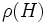# Direct factor over central subgroup

## Contents

BEWARE! This term is nonstandard and is being used locally within the wiki. [SHOW MORE]
This article defines a subgroup property: a property that can be evaluated to true/false given a group and a subgroup thereof, invariant under subgroup equivalence. View a complete list of subgroup properties[SHOW MORE]

## Definition

### Definition with symbols

Suppose$H$ is a subgroup of a group$G$. We say that$H$ is a direct factor over central subgroup of$G$ if it satisfies the following equivalent conditions:

1. There exists a subgroup$A$ of$H$ such that$A$ is a central subgroup of$G$ and$H/A$ is a direct factor of the quotient group$G/A$.
2. In the quotient map$\rho:G \to G/Z(G)$, where$Z(G)$ is the center of$G$,$\rho(H)$ is a direct factor of$\rho(G) = G/Z(G)$.

## Relation with other properties

### Stronger properties

property quick description proof of implication proof of strictness (reverse implication failure) intermediate notions
Direct factor Join of direct factor and central subgroup|FULL LIST, MORE INFO
Central subgroup Join of direct factor and central subgroup|FULL LIST, MORE INFO